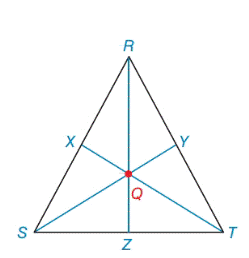Chapter 7.2, Problem 27E### Elementary Geometry for College St...

6th Edition
Daniel C. Alexander + 1 other
ISBN: 9781285195698

#### Solutions

Chapter
Section### Elementary Geometry for College St...

6th Edition
Daniel C. Alexander + 1 other
ISBN: 9781285195698
Textbook Problem
38 views

# Given: Isosceles Δ R S T R S = R T = 17         a n d         S T = 16. Medians R Z ¯ , T X ¯ and S Y ¯ meet at centroid Q .Find: R Q         and           S QExercises 29, 30

To determine

To find:

The RQandSQ in the given isosceles triangle RST.

Explanation

Definition:

The three medians of a triangle are congruent at a point that is two-thirds the distance from any vertex to the midpoint of the opposite side.

Formula used:

The triangle RST, with sides a,bandc opposite vertices R,SandT. Then the length of the median ma form R is given by

ma=122(b2+c2)a2

The length of the median mb form S is given by

mb=122(c2+a2)b2

Where ma,mb denotes the length of the median

Given:

In ΔRST, medians RZ¯, TX¯ and SY¯ intersect at centroid Q. If RS=RT=17andST=16.

Calculation:

The point of concurrence Q for the three medians of a triangle is known as centroid of the triangle. In ΔMNP, medians MB¯, NA¯ and PC¯ intersect at centroid Q.

According to the definition,

RQ=23(RZ)(1)SQ=23(SY)(2)

Given RS=RT=17andST=16.

The triangle RST, with sides a,bandc opposite vertices R,SandT.

So, ST=16=a,RT=17=bandRS=17=c.

We sketch the figure of the isosceles triangle RST with sides a,bandc

First we need to find length of the median RZ,

Using the above formula,

The length of the median ma form R is given by

RZ=ma=122(b2+c2)a2=122(172+172)162=122(289+289)256=122(578)256=121156256=12900

### Still sussing out bartleby?

Check out a sample textbook solution.

See a sample solution

#### The Solution to Your Study Problems

Bartleby provides explanations to thousands of textbook problems written by our experts, many with advanced degrees!

Get Started

#### Evaluate limxesinx1x.

Single Variable Calculus: Early Transcendentals, Volume I

#### Evaluate the integrals in Problems 7-36. Check your results by differentiation. 8.

Mathematical Applications for the Management, Life, and Social Sciences

#### Find for x = 3t2 + 1, y = t6 + 6t5. t4 + 5t3 4t3 + 15t2

Study Guide for Stewart's Multivariable Calculus, 8th

#### In Exercises 23-30, use logarithms to solve the equation for t. e0.4t=8

Finite Mathematics for the Managerial, Life, and Social Sciences

#### ∫x−4dx = −4x−5 + C

Study Guide for Stewart's Single Variable Calculus: Early Transcendentals, 8th

#### Explain why plagiarism is unethical.

Research Methods for the Behavioral Sciences (MindTap Course List)